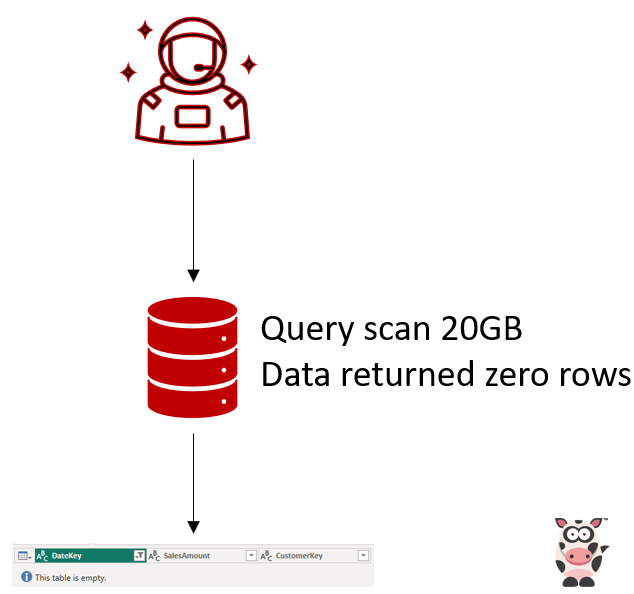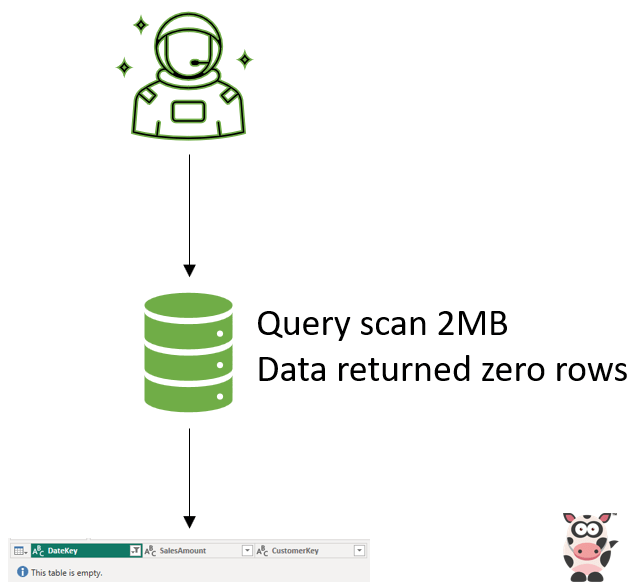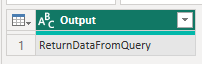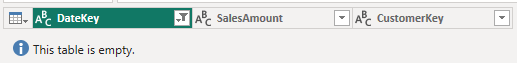I had a requirement where I wanted to only have the underlying query run if there was data in the table.

As shown in the image below a query would run, scan 20GB and then return zero rows.After making the changes what then happens is an initial query runs which will count the number of rows and if there is 1 row or more then run the underlying query, otherwise return a blank table.

By doing it in this manner I can save 20GB of scan costs an SQL Serverless each time the dataset refreshes. Here is an example of what this change looks like below when there is no data returned.The reason for this is so that if there is no data then do not run a query which will have to scan all the data to complete and then still return 0 rows.

When using SQL Serverless where you pay for how much data you scan per query, this reduces the amount of data scanned.

If you then had to be refreshing the dataset 24 times a day this can mean significant cost savings!

NOTE: The important thing here was that the logic runs because based on the output of the first query it will determine which query to run next.

Here is the code below how I got this working. I did test this, and it does indeed work!

```let

// This is to check if there is any data being returned.

// Just Count the Number of Rows to determine if there is any data.

Source = Sql.Database("sqlserverless.sql.azuresynapse.net", "Parquet_Datasets", [Query="

Select count(1) as TotalRows

From dbo.tb_My_FactTable

Where DateKey = 20230204

"),

// This step returns a single value back which can then be used in the later steps.

WhichQueryToRun = #"Source"{0}[TotalRows],

// The first part of the if condition is if there is data then run this query below.

ActualSteps = if WhichQueryToRun >= 1 then

Sql.Database("sqlserverless.sql.azuresynapse.net", "Parquet_Datasets", [Query="

Select

DateKey,

SalesAmount = SUM(Sales_Values),

CustomerKey

From dbo.tb_My_FactTable

Where DateKey = 20230204

")

// If there is no data then return a blank table.

else Sql.Database("sqlserverless.sql.azuresynapse.net", "Parquet_Datasets", [Query="

Select

null as DateKey ,

null as SalesAmount,

null as CustomerKey

"),

// The reason I filter out the null values is to ensure that the table is empty and has NO null values.

#"Filtered Rows" = Table.SelectRows(ActualSteps, each [DateKey] <> null)

in

#"Filtered Rows"```

This is the result below if there are zero rows.And then if there is data this is what it will return.Thanks for reading and I hope that you found this blog post useful and something you can use in your datasets.